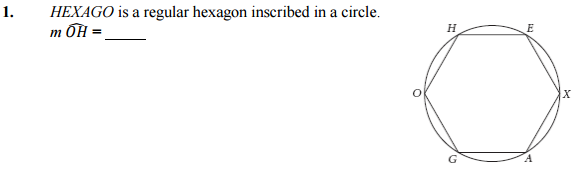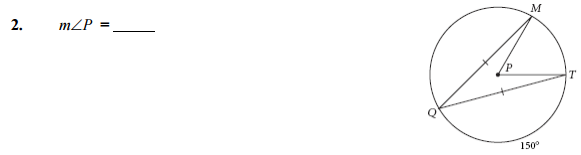# Chord Properties

Topic:
Circle

## Lesson 9.2 • Chord Properties In this lesson you will discover some properties of chords, arcs, and central angles. Investigation 1: Chords and Their Central Angles

1. Drag different parts of your figure to confirm that the chords you constructed stay congruent. Measure central angles CAB and DAE. (Remember, to measure an angle, make sure the vertex is the second point you select.) Write a conjecture about congruent chords in a circle and the central angles they determine (Chord Central Angles Conjecture). 2. The measure of a minor arc is defined as the measure of its central angle. Measure the arc to confirm this. Measure the arc intercepted by the other chord. What can you conclude about congruent chords in a circle and the arcs they intercept? Write a conjecture (Chord Arcs Conjecture).

Chord Central Angles Conjecture: If two chords in a circle are congruent, then they determine two central angles that are ___________.

Chord Arcs Conjecture: If two chords in a circle are congruent, then their _________________ are congruent.

Prove the statement "If arc ED is congruent to arc CB, then segment ED is congruent to segment CB" Hint: Use the fact that if arcs are congruent, their central angles are congruent. Also, remember, that all radii of a circle are congruent.